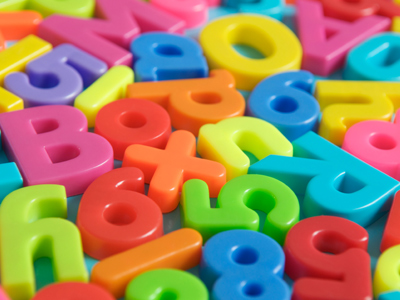Test your math skills in this quiz.

# Algebra - Putting Numbers in Place of Letters

This Math quiz is called 'Algebra - Putting Numbers in Place of Letters' and it has been written by teachers to help you if you are studying the subject at middle school. Playing educational quizzes is a fabulous way to learn if you are in the 6th, 7th or 8th grade - aged 11 to 14.

It costs only \$12.50 per month to play this quiz and over 3,500 others that help you with your school work. You can subscribe on the page at Join Us

In algebra, letters, such as a and b, are used in place of numbers. If the values of the letters are known then it is important to be able to replace the letters with numbers.

This Math quiz will give you plenty of practice in the subject.

1.
If x = 9 and y = 23, what is the value of y - x?
14
16
18
20
23 - 9 = 14
2.
If a = 4 and b = 2, what is the value of a2 / b?
8
16
4
2
16 / 2 = 8
3.
If x = 5 and y = 10, what is the value of xy / 10
3
5
10
20
Two letters together need to be multiplied together
4.
If a = 2 and b = 3, what is the value of a + b + a?
5
6
7
8
2 + 3 + 2 = 7
5.
If x = 6 and y = 7, which of the following gives a value of 55
x2 + y2
xy2
x + y2
2x + 2y
6.
If a = 6 and x = 8, which of the following gives a value of 22?
a + x2
a + 2x
2a + 2x
2ax
'If in doubt, write it down and work it out'
7.
If a = 2, b = 3, c = 4 and d = 5, what is the value of 2a + 3b + c2 - d
24
26
28
30
4 + 9 + 16 - 5 = 24. Don't forget 'IF IN DOUBT, WRITE IT DOWN AND WORK IT OUT!
8.
If a = 4 and b = 5, what is the value of 2a + b
7
9
11
13
Remember that when a number and a letter are together they need to be multiplied together
9.
If a = 4 and b = 8, what is the value of 2a + x2
64
72
80
It is impossible to say
Notice that we did not give you a value for x and therefore you cannot work out the expression!
10.
If x = 2 and b = 4, what is the value of x2 + b2?
12
16
20
24
(2 x 2) + (4 x 4)
Author:  Frank Evans